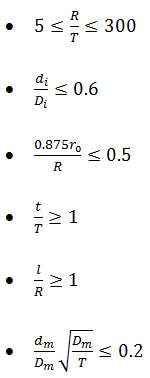#### Introduction to WRC 107: Concepts, Limitations and Formula

Welding research council bulletin 107 which is globally known as WRC-107 introduces one of well-known and important methods for calculation of local stresses in junction of shell and nozzle. This method was developed by Professor B.P.Bijlaard and firstly published by WRC in 1965. Some well-known softwares such as CAESAR II, PVElit and Compress use this method for nozzle loads checking. There are some important items that engineers who use these softwares should be aware. Its limitations, scope and concepts of local stress cause are mentioned here. WRC-107 consists of many curves enabling engineers to calculate all types of local stresses caused by external loads exerting on a circular or rectangular nozzle on shell elements. The nozzle is assumed to be penetrated to a cylindrical or spherical shell.

As shown in figure 1, local stresses are calculated at 8 points, named as                                    ,  located on two levels, upper and lower plates. The local stresses are calculated by some geometric parameters which can be extracted by curves. For making a proper stress combination according to stress categories introduced in ASME BPVC Section VIII Div 2, an engineer should know the types of all local stresses and their causes.Figure 1: 6 external loads and 8 points on which local stresses are studied in WRC-107

1- Local stress types due to external loads

WRC-107 supports 6 external loads in local stress calculation: Axial force, two lateral force, torsional moment and two bending moments as shown in figure 1. Each load creates some types of local stresses such as membrane or bending stresses in two directions: longitudinal and circumferential. Depending on other applied loadings such as system weight, internal or external pressure, wind or earthquake, snow, thermal loads and etc., each type of mentioned local stresses should be combined with their relative stresses in a proper way. The outcome may lead to under design or overdesign If piping stress engineers or pressure vessel engineers who take care of nozzle loads, do not know how to treat all extracted local stresses properly. For overcoming this common problem, engineers must have knowledge about firstly all types of local stresses and secondly stress combination methods which have been introduced by ASME BPVC Section VIII Div 2. Sign of stresses are important in the stress combination.

1-1-Local stresses due to axial force, PFigure 2: Longitudinal and circumferential membrane stresses due to an external axial loadFigure 3: Longitudinal and circumferential bending stresses due to an external axial load

1-1-1-Membrane stress

Axial force, P, pushes the joint lines on which mentioned 8 points are placed in such a way a resistant force comes up. As shown in figure 2, the joint line tends to move in both longitudinal and circumferential directions but because of the resistance, two forces            appear.Users can refer to proper curves to extract                  by help of geometric parameters.1-1-2-Bending stress

Axial load bend the junction line locally as shown in figure 3 and creates two bending moments at each 8 points: one in circumferential direction,      , and the other in longitudinal direction,     .The mentioned bending stresses which are defined as                    can be extracted by proper curves.1-2-Local stresses due to longitudinal bending momentFigure 4: Longitudinal and circumferential membrane stresses due to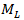Figure 5: Longitudinal and circumferential bending stresses due to1-2-1-Membrane stress

Referring to figure 4,      tends to push point A and pull point B. So, because of existing resistance against them, two forces appear: one in longitudinal and another in circumferential direction. Actually these forces stem from resistance of the adjacent area against the pushing and pulling. These forces which are shown in figure 4 as       create membrane stresses named as                which can be calculated based on                      from suitable curves.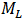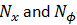1-2-2-Bending Stress

As shown in figure 5, the longitudinal bending moment bends edges A and B locally in such away two bending moments are created at the points A and B: a longitudinal bending moment,     , and a circumferential bending moment,     . The relative stresses are defined as                   they are calculated by                     which are extracted by proper curves.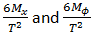1-3-Local stresses due to external circumferential bending moment

The circumferential bending moment creates membrane and bending stresses at points C and D in a similar way described in section 1-2: “longitudinal bending moment” part. For calculating the mentioned stresses,     should be substituted by      and proper curves must be used.1-4-Shear stresses resulting from external torsional moment (       ) and external lateral forcesFigure 6: Shear stresses

The lateral forces including longitudinal and circumferential, named as          respectively, as well as torsional moment named as      produce shear stresses which can be calculated based on the formula in WRC-107. These shear stresses are clearly shown in figure 6.1-5-Stress calculation summary table

The table 1 can be used to calculate the mentioned stresses when using WRC-107. In this table, all required stress elements and their curves number are addressed for points                       . The direction (longitudinal/ circumferential), category (membrane/ bending) and the source load are clearly mentioned in the table.Table 1: Local stresses stemming from 6 external loads with stress sources

2-Assumptions and limitations

WRC-107 is not applicable for all cases. Actually, because of some limitations blind use of WRC-107 may lead to a dangerous design. Therefore engineers who use this method must be familiar with the restrictions. Some of these limitations result from geometrical parameters which are used in the curves or the areas which are covered by them. Some of these limitations are as follows:

• WRC-107 is limited to stresses on shells not on nozzles. So, this method cannot be used for cases in which maximum stress takes place on a nozzle instead of a shell. It maybe happens when the nozzle is not strong enough comparing to the shell and so there is a possibility that the maximum stress goes to nozzle. In this case WRC-297 maybe used instead.

• When stiffness of a connecting pipe differs significantly from shell at the nozzle-shell junction, depending on this difference, elastic characteristic of system and temperature difference, a plastic failure at the part of system which has smaller stiffness may take place.  Strain concentration factor is used and calculated to show this possibility. WRC-107 says nothing about this factor. Finite element software such as Nozzle Pro or FE Pipe give the factor in their results.